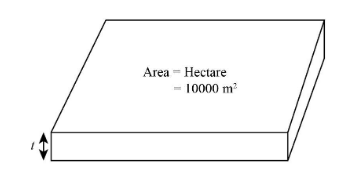QuestionAnswers

# Half of the cubic meter of the gold sheet is extended by hammering so as to cover an area of 1 hectare. The thickness of the sheet is:A. ${\text{0}}{\text{.0005}}\;{\text{cm}}$ B. ${\text{0}}{\text{.005}}\;{\text{cm}}$ C. ${\text{0}}{\text{.05}}\;{\text{cm}}$ D. ${\text{0}}{\text{.5}}\;{\text{cm}}$Verified
92.4k+ views
Hint: To find the thickness of the gold sheet, we will divide the volume of the gold sheet by its area of the cross section. The sheet is of the shape of a cuboid, so we can apply the formula of volume of a cuboid, which is given as:
$v = l \times b \times t$
Where, $l$ is the length , $b$ is the breadth and $t$ is the thickness of the cuboid. The area of the cross section of the cuboid can be expressed as $l \times b$ . We will use these expressions to find the thickness.

Given:
Volume of the gold sheet is $v = 0.5\;{{\text{m}}^3}$ .
Area of the gold sheet is $A = 1\;{\text{hectare}}$ .
The following is the schematic diagram of an extended gold cube.The expression for the volume of gold sheet will be
$v = l \times b \times t$
Where $l$ is the length, $b$ is the breadth and $t$ is the thickness of the gold sheet.
Also, area of the cross section on which are hammering can be expressed as:
$A = l \times b$
Hence, we can find the thickness of the sheet by dividing volume of the gold sheet by the area of the gold sheet which can be expressed as:
$t = \dfrac{v}{A}$
Now, we will substitute $1\;{\text{hectare}}$ for $A$ and $0.5\;{{\text{m}}^3}$ for $v$ in the above expression.
$\begin{array}{l} t = \dfrac{{0.5\;{{\text{m}}^{\text{3}}}}}{{1\;{\text{hectare}} \times \dfrac{{{\text{10000}}\;{{\text{m}}^{\text{2}}}}}{{{\text{1}}\;{\text{hectare}}}}}}\\ t = \dfrac{5}{{100000}}\;{\text{m}}\\ t = 0.000005\;{\text{m}} \times \dfrac{{{\text{1}}{{\text{0}}^{\text{2}}}\;{\text{cm}}}}{{{\text{1}}\;{\text{m}}}}\\ t = 0.0005\;{\text{cm}} \end{array}$
So, the correct answer is “Option A”.

Note: The area of the cross section of the gold sheet is given in hectares. So it has to be converted into meter squares for the calculation. But the options are in centimetre, so we will again convert the result into centimetre. The calculation should always be done in the standard units only.